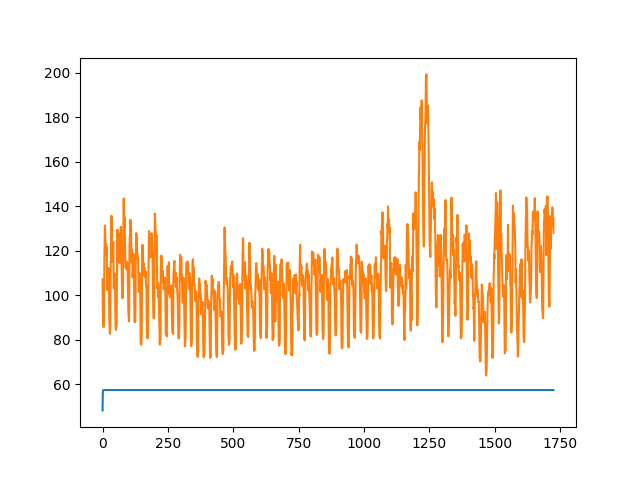# All prediction values identical when using model for inference

I am using a saved model for inference. When I used it to predict values, the values converge very quickly and the majority are predicted as the same value. This is even though my input dataset has significant variability. The loss decreases when training.

I am not sure why this is happening, any suggestions would be much appreciated!

Here is my code and some sample input data and output data:

``````model_state = torch.load(model_path)
model = LSTMModel(model_state['input_dim'], model_state['hidden_dim'],
model_state['num_layers'], model_state['output_dim'])
for parameter in model.parameters():

model.eval()

num_features = (len(data.columns)-1)
actual_data = torch.tensor(data[target_col].values).float()
prediction_data = torch.tensor(data.drop(columns=target_col).values).float()
actual_data = actual_data.view(-1, 1, 1)
prediction_data = prediction_data.view(-1, 1, num_features)

prediction = model(prediction_data)
prediction = prediction.data.numpy()
``````

Input Data:

Output predictions:

Have you seen the same effect during validation?
Do you handle the validation and test set in the same manner, i.e. regarding the initial hidden state etc.?

Yes I am seeing the same effect in validation and I am handling both sets in the same manner.
For clarity my LSTM has 10 neurons per layer, 1 hidden layer, a learning rate of 0.001 and 20000 training epochs. Might it be that my network is not deep enough?Attached is a figure showing the output I am receiving.
The orange line is the actual data and the blue line is my forecast.